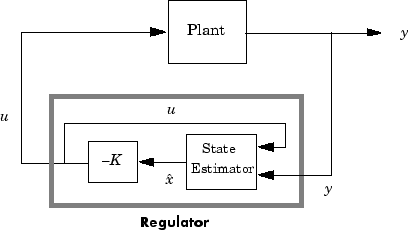reg

Form regulator given state-feedback and estimator gains

Syntax

rsys = reg(sys,K,L)
rsys = reg(sys,K,L,sensors,known,controls)

Description

rsys = reg(sys,K,L) forms a dynamic regulator or compensator rsys given a state-space model sys of the plant, a state-feedback gain matrix K, and an estimator gain matrix L. The gains K and L are typically designed using pole placement or LQG techniques. The function reg handles both continuous- and discrete-time cases.

This syntax assumes that all inputs of sys are controls, and all outputs are measured. The regulator rsys is obtained by connecting the state-feedback law u = –Kx and the state estimator with gain matrix L (see estim). For a plant with equations

$\begin{array}{l}\stackrel{˙}{x}=Ax+Bu\\ y=Cx+Du\end{array}$

this yields the regulator

$\begin{array}{l}\stackrel{˙}{\stackrel{^}{x}}=\left[A-LC-\left(B-LD\right)K\right]\stackrel{^}{x}+Ly\\ u=-K\stackrel{^}{x}\end{array}$

This regulator should be connected to the plant using positive feedback.rsys = reg(sys,K,L,sensors,known,controls) handles more general regulation problems where:

• The plant inputs consist of controls u, known inputs ud, and stochastic inputs w.

• Only a subset y of the plant outputs is measured.

The index vectors sensors, known, and controls specify y, ud, and u as subsets of the outputs and inputs of sys. The resulting regulator uses [ud ; y] as inputs to generate the commands u (see next figure).Examples

Given a continuous-time state-space model

sys = ss(A,B,C,D)

with seven outputs and four inputs, suppose you have designed:

• A state-feedback controller gain K using inputs 1, 2, and 4 of the plant as control inputs

• A state estimator with gain L using outputs 4, 7, and 1 of the plant as sensors, and input 3 of the plant as an additional known input

You can then connect the controller and estimator and form the complete regulation system by

controls = [1,2,4];
sensors = [4,7,1];
known = ;
regulator = reg(sys,K,L,sensors,known,controls)